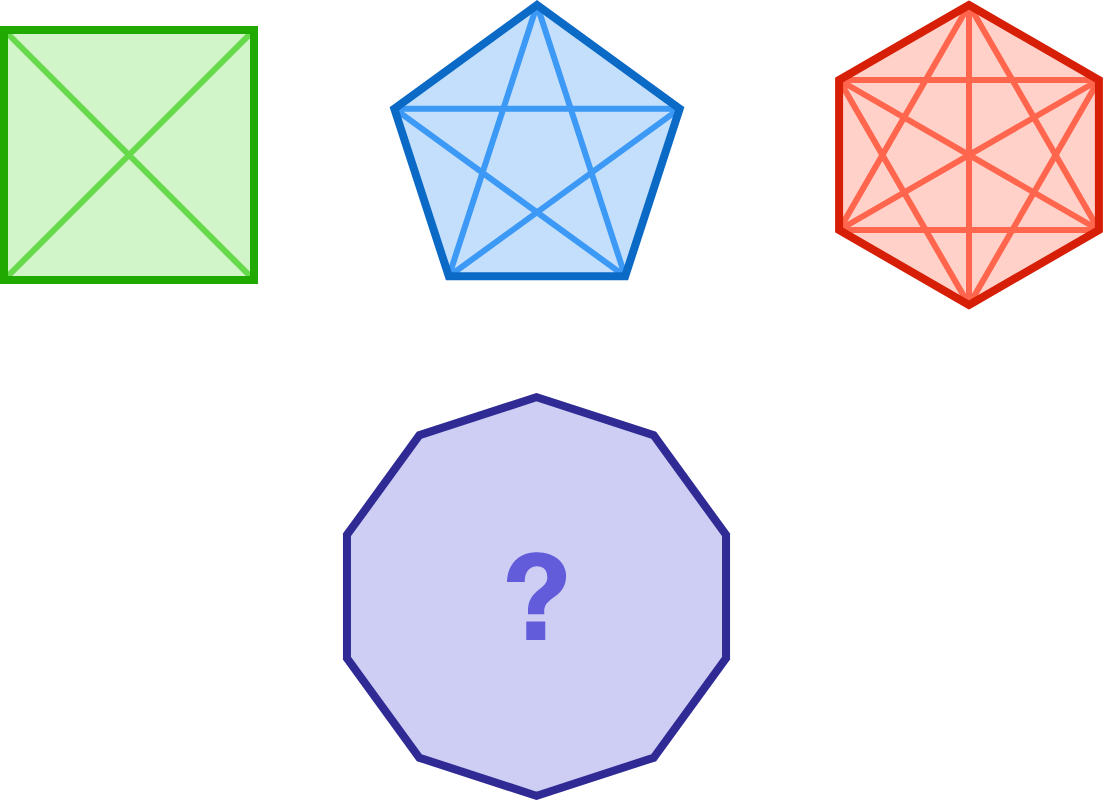# Daring Diagonals

Probability Level 1

A convex quadrilateral has 2 diagonals. A convex pentagon has 5 diagonals. A convex hexagon has 9 diagonals.

How many diagonals does a convex decagon (10 sides) have?×